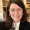Develop an assignment that assesses a student's ability to "solve first-order ordinary differential equations using techniques such as separation of variables, integrating factors, and substitution methods."

Assignment: Solving First-Order Ordinary Differential Equations

Instructions:

1. Given the following differential equation, use separation of variables to find the general solution: dy/dx = 2x/y

2. Given the following differential equation, use an integrating factor to find the general solution: (2x + 3y) dy/dx = 6x^2 - 9y^2

3. Given the following differential equation, use the substitution method to find the general solution: dy/dx + 2y = 3e^x

4. Given the following differential equation, use any method of your choice to find the general solution: (x^2 + y^2) dy/dx = xy^2

5. Finally, given the general solution to each differential equation, use it to find the particular solution that satisfies the initial condition: y(0) = 1 for the first equation y(1) = 2 for the second equation y(0) = 1 for the third equation y(1) = 2 for the fourth equation